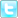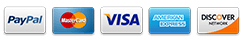# Thermodynamics and Heat Engines

Thermodynamics is a branch of physics that deals with the relationships and conversions of heat into other forms of energy. There are four fundamental laws of thermodynamics that defines thermodynamic properties and explains the functionality of a physical system. They are: Zeroth law that deals with thermal equilibrium, first law that deals with conservation of energy, second law that deals with entropy, and third law that deals with absolute zero of temperature.

The heat engine operates on a thermodynamic cycle. A heat engine is a device that converts heat energy into work or mechanical energy. The heat engines use first law and second law of thermodynamics to convert heat into work. Heat engines can be studied using the pressure-volume (PV) diagrams or the reservoir model.

Boyle's Law and Charles's Law

Boyle’s Law: Boyle’s law is an ideal gas law, which states that at a constant temperature and quantity, the volume of an ideal gas is inversely proportional to the pressure on the gas. This law is named after physicist and chemist Robert Boyle.

The formula for Boyle’s law is P α 1/V or PV = constant where P is the pressure on the gas and V is the volume of the gas.

Charles’s Law: Charles’s law is also an ideal gas law and it states that under constant temperature and quantity, the volume of an ideal gas is directly proportional to the temperature of the gas.

The formula for Charles’s law is V α T or V/ T = constant where V is the volume of the gas and
T is the temperature of the gas.

• Boyle’s Law: Here, Boyle’ law is clearly explained with an experiment and a simple example.
• Charles’s Law: This site provides good explanation, background, discussions, and calculations on the Charles’s law.

The First Law of Thermodynamics

The first law of thermodynamics deals with the processes of thermodynamics and conservation of energy. The key concepts of the first law are heat, internal energy, and system work. The first law states that the energy cannot be created or destroyed, but it can be transformed from one form to another. Alternatively, the first law is also defined as the change in the internal energy of the thermodynamic system is equal to the amount of heat added to the system minus the amount lost due to the work performed by the system.

The formula for the first law of thermodynamics is ?U = Q-W where ?U is the change in the internal energy, Q is the heat added and W is the work done.

• First Law of Thermodynamics: Here, definition, key concepts, and formulas of the first law are clearly explained.
• The First Law: Here, definition, formula, example, and the pressure-volume graph of the first law are explained. Also, various types of thermodynamic processes are given with PV graph.

Second Law of Thermodynamics

The second law of thermodynamics is the law of increased entropy, and it states that in every process, the entropy of the isolated system either increases or remains constant. The entropy is the fundamental concept of the system and it is the heat absorbed to the temperature of the system. The entropy is represented as S and the symbol ?S is the change in the entropy in the thermodynamic system.

The formula for the increased entropy is given as ?S = Q \ T where Q is the heat absorbed and T is the temperature.

• The Second Law of Thermodynamics: Here, definition of second law and entropy, and evolution and probability of second law are clearly given with formulas.
• Entropy and the Second Law: This site provides clear explanation on entropy, the second law, and the relationship between entropy and energy in the system.

Heat Engines

A heat engine is a cyclic process that absorbs energy in the form of heat to perform mechanical work and then expels the heat that cannot be used to do any work. The efficiency of a heat engine is given as the amount of useful work done to the amount of the heat energy supplied to the system. The first law and second law of thermodynamics restrain the function of a heat engine. The first law determines the conservation of energy and the second law determines the flow of energy. The heat engines are commonly defined by a PV diagram.

The efficiency of a heat engine is 1 – (Qc/Qh) where Qc is the exhausted heat energy and Qh is the absorbed heat energy.

• Heat Engines: This site provides a quick introduction to the heat engines, various formulas, and the basic concepts of the heat engines.
• Heat Engines and the Carnot Cycle: Here, the concept of a heat engine is explained along with the Carnot cycle with a neat PV diagram.

Written By: | Email |Popular Pages

Popular Model Pages

Popular ArticlesBROWSE Home View Cart Catalog Articles
SECURITY